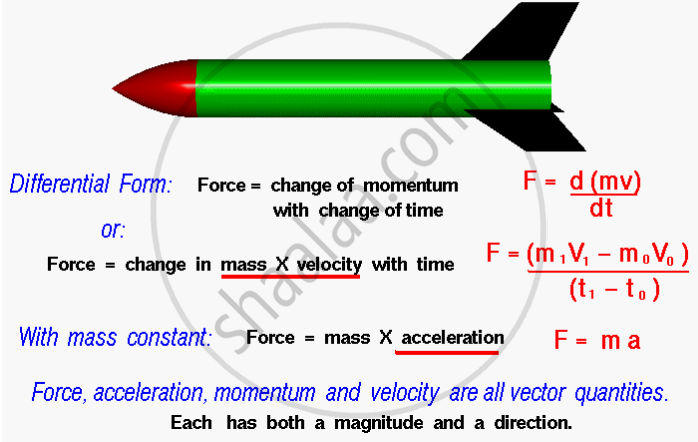Share

# Second Law of Motion

### Second Law of Motion

“The rate of change of momentum of an object is proportional to the applied unbalanced force in the direction of the force.”This law of motion is also called the Law of Force and Acceleration. It means that force is equal to the change in momentum per change in time. For a constant mass, force equals mass times acceleration.

### Shaalaa.com

Newton's 2nd second law, Momentum [00:21:02]
S
series 1
0%

S